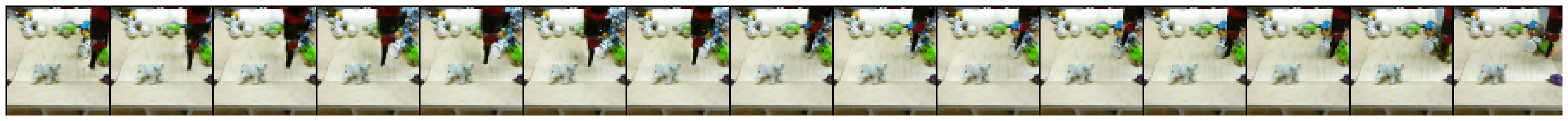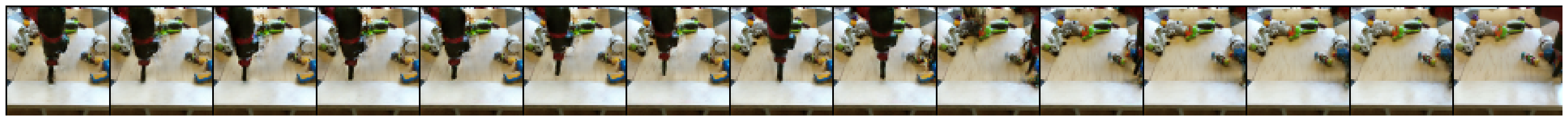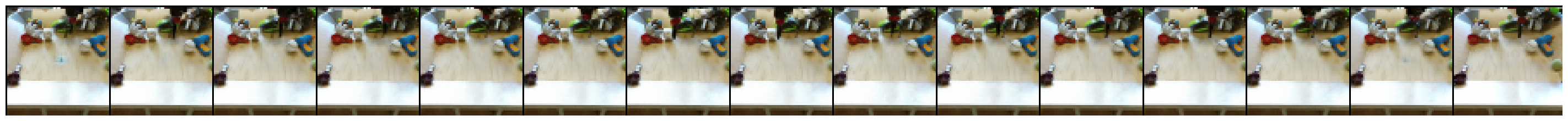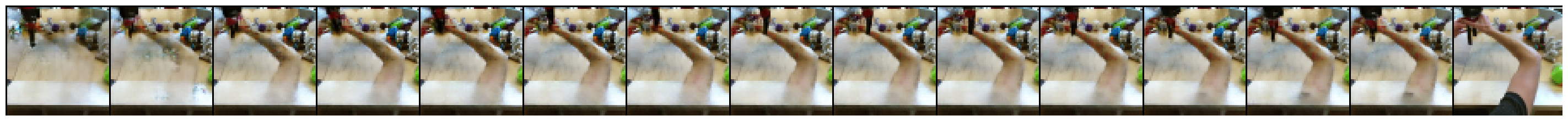Save the date! Google I/O returns May 18-20

# 使用 3D 卷积实现视频插画

Yunpeng Li、Dominik Roblek 和 Marco Tagliasacchi。From Here to There: Video Inbetweening Using Direct 3D Convolutions，2019 年。

https://arxiv.org/abs/1905.10240

• 具有适用于 BAIR 机器人推动视频和 KTH 动作视频数据集的模型（不过，本 Colab 仅使用 BAIR）
• Hub 中已提供 BAIR 数据集，但需要用户自己提供 KTH 视频。
• 目前仅限评估（视频生成）
• 批次大小和帧大小为硬编码

## 设置

import tensorflow as tf

import matplotlib.pyplot as plt
import numpy as np
import seaborn as sns
import tensorflow_hub as hub
import tensorflow_datasets as tfds

from tensorflow_datasets.core import SplitGenerator
from tensorflow_datasets.video.bair_robot_pushing import BairRobotPushingSmall

import tempfile
import pathlib

TEST_DIR = pathlib.Path(tempfile.mkdtemp()) / "bair_robot_pushing_small/softmotion30_44k/test/"

# Download the test split to $TEST_DIR mkdir -p$TEST_DIR
wget -nv https://storage.googleapis.com/download.tensorflow.org/data/bair_test_traj_0_to_255.tfrecords -O \$TEST_DIR/traj_0_to_255.tfrecords

2020-11-12 08:05:31 URL:https://storage.googleapis.com/download.tensorflow.org/data/bair_test_traj_0_to_255.tfrecords [189852160/189852160] -> "/tmp/tmpom9dguvr/bair_robot_pushing_small/softmotion30_44k/test/traj_0_to_255.tfrecords" 

# Since the dataset builder expects the train and test split to be downloaded,
# patch it so it only expects the test data to be available
builder = BairRobotPushingSmall()
test_generator = SplitGenerator(name='test', gen_kwargs={"filedir": str(TEST_DIR)})
builder._split_generators = lambda _: [test_generator]
builder.download_and_prepare()

Downloading and preparing dataset bair_robot_pushing_small/2.0.0 (download: 30.06 GiB, generated: Unknown size, total: 30.06 GiB) to /home/kbuilder/tensorflow_datasets/bair_robot_pushing_small/2.0.0...
WARNING:tensorflow:From /home/kbuilder/.local/lib/python3.6/site-packages/tensorflow_datasets/video/bair_robot_pushing.py:103: tf_record_iterator (from tensorflow.python.lib.io.tf_record) is deprecated and will be removed in a future version.
Instructions for updating:
Use eager execution and:
tf.data.TFRecordDataset(path)
WARNING:tensorflow:From /home/kbuilder/.local/lib/python3.6/site-packages/tensorflow_datasets/video/bair_robot_pushing.py:103: tf_record_iterator (from tensorflow.python.lib.io.tf_record) is deprecated and will be removed in a future version.
Instructions for updating:
Use eager execution and:
tf.data.TFRecordDataset(path)
Shuffling and writing examples to /home/kbuilder/tensorflow_datasets/bair_robot_pushing_small/2.0.0.incompleteB1XZUH/bair_robot_pushing_small-test.tfrecord
Dataset bair_robot_pushing_small downloaded and prepared to /home/kbuilder/tensorflow_datasets/bair_robot_pushing_small/2.0.0. Subsequent calls will reuse this data.


## BAIR：基于 NumPy 数组输入的演示

WARNING:tensorflow:From /home/kbuilder/.local/lib/python3.6/site-packages/tensorflow_datasets/core/features/feature.py:425: calling map_fn_v2 (from tensorflow.python.ops.map_fn) with back_prop=False is deprecated and will be removed in a future version.
Instructions for updating:
back_prop=False is deprecated. Consider using tf.stop_gradient instead.
Instead of:
results = tf.map_fn(fn, elems, back_prop=False)
Use:
results = tf.nest.map_structure(tf.stop_gradient, tf.map_fn(fn, elems))
WARNING:tensorflow:From /home/kbuilder/.local/lib/python3.6/site-packages/tensorflow_datasets/core/features/feature.py:425: calling map_fn_v2 (from tensorflow.python.ops.map_fn) with back_prop=False is deprecated and will be removed in a future version.
Instructions for updating:
back_prop=False is deprecated. Consider using tf.stop_gradient instead.
Instead of:
results = tf.map_fn(fn, elems, back_prop=False)
Use:
results = tf.nest.map_structure(tf.stop_gradient, tf.map_fn(fn, elems))


Test videos shape [batch_size, start/end frame, height, width, num_channels]:  (16, 2, 64, 64, 3)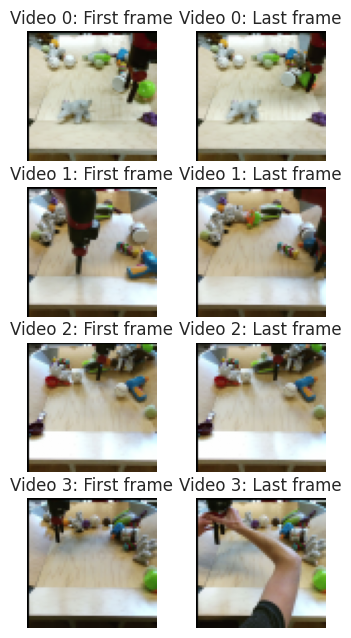### 加载 Hub 模块

hub_handle = 'https://hub.tensorflow.google.cn/google/tweening_conv3d_bair/1'
module = hub.load(hub_handle).signatures['default']

WARNING:tensorflow:Unable to create a python object for variable <tf.Variable 'video_discriminator/conv_0/weights:0' shape=(4, 4, 4, 3, 64) dtype=float32_ref> because it is a reference variable. It may not be visible to training APIs. If this is a problem, consider rebuilding the SavedModel after running tf.compat.v1.enable_resource_variables().
WARNING:tensorflow:Unable to create a python object for variable <tf.Variable 'video_discriminator/conv_0/weights:0' shape=(4, 4, 4, 3, 64) dtype=float32_ref> because it is a reference variable. It may not be visible to training APIs. If this is a problem, consider rebuilding the SavedModel after running tf.compat.v1.enable_resource_variables().
WARNING:tensorflow:Unable to create a python object for variable <tf.Variable 'video_discriminator/conv_0/LayerNorm/beta:0' shape=(64,) dtype=float32_ref> because it is a reference variable. It may not be visible to training APIs. If this is a problem, consider rebuilding the SavedModel after running tf.compat.v1.enable_resource_variables().
WARNING:tensorflow:Unable to create a python object for variable <tf.Variable 'video_discriminator/conv_0/LayerNorm/beta:0' shape=(64,) dtype=float32_ref> because it is a reference variable. It may not be visible to training APIs. If this is a problem, consider rebuilding the SavedModel after running tf.compat.v1.enable_resource_variables().
WARNING:tensorflow:Unable to create a python object for variable <tf.Variable 'video_discriminator/conv_0/LayerNorm/gamma:0' shape=(64,) dtype=float32_ref> because it is a reference variable. It may not be visible to training APIs. If this is a problem, consider rebuilding the SavedModel after running tf.compat.v1.enable_resource_variables().
WARNING:tensorflow:Unable to create a python object for variable <tf.Variable 'video_discriminator/conv_0/LayerNorm/gamma:0' shape=(64,) dtype=float32_ref> because it is a reference variable. It may not be visible to training APIs. If this is a problem, consider rebuilding the SavedModel after running tf.compat.v1.enable_resource_variables().
WARNING:tensorflow:Unable to create a python object for variable <tf.Variable 'video_discriminator/conv_1/weights:0' shape=(4, 4, 4, 64, 128) dtype=float32_ref> because it is a reference variable. It may not be visible to training APIs. If this is a problem, consider rebuilding the SavedModel after running tf.compat.v1.enable_resource_variables().
WARNING:tensorflow:Unable to create a python object for variable <tf.Variable 'video_discriminator/conv_1/weights:0' shape=(4, 4, 4, 64, 128) dtype=float32_ref> because it is a reference variable. It may not be visible to training APIs. If this is a problem, consider rebuilding the SavedModel after running tf.compat.v1.enable_resource_variables().
WARNING:tensorflow:Unable to create a python object for variable <tf.Variable 'video_discriminator/conv_1/LayerNorm/beta:0' shape=(128,) dtype=float32_ref> because it is a reference variable. It may not be visible to training APIs. If this is a problem, consider rebuilding the SavedModel after running tf.compat.v1.enable_resource_variables().
WARNING:tensorflow:Unable to create a python object for variable <tf.Variable 'video_discriminator/conv_1/LayerNorm/beta:0' shape=(128,) dtype=float32_ref> because it is a reference variable. It may not be visible to training APIs. If this is a problem, consider rebuilding the SavedModel after running tf.compat.v1.enable_resource_variables().
WARNING:tensorflow:Unable to create a python object for variable <tf.Variable 'video_discriminator/conv_0/weights:0' shape=(4, 4, 4, 3, 64) dtype=float32_ref> because it is a reference variable. It may not be visible to training APIs. If this is a problem, consider rebuilding the SavedModel after running tf.compat.v1.enable_resource_variables().
WARNING:tensorflow:Unable to create a python object for variable <tf.Variable 'video_discriminator/conv_0/weights:0' shape=(4, 4, 4, 3, 64) dtype=float32_ref> because it is a reference variable. It may not be visible to training APIs. If this is a problem, consider rebuilding the SavedModel after running tf.compat.v1.enable_resource_variables().
WARNING:tensorflow:Unable to create a python object for variable <tf.Variable 'video_discriminator/conv_0/LayerNorm/beta:0' shape=(64,) dtype=float32_ref> because it is a reference variable. It may not be visible to training APIs. If this is a problem, consider rebuilding the SavedModel after running tf.compat.v1.enable_resource_variables().
WARNING:tensorflow:Unable to create a python object for variable <tf.Variable 'video_discriminator/conv_0/LayerNorm/beta:0' shape=(64,) dtype=float32_ref> because it is a reference variable. It may not be visible to training APIs. If this is a problem, consider rebuilding the SavedModel after running tf.compat.v1.enable_resource_variables().
WARNING:tensorflow:Unable to create a python object for variable <tf.Variable 'video_discriminator/conv_0/LayerNorm/gamma:0' shape=(64,) dtype=float32_ref> because it is a reference variable. It may not be visible to training APIs. If this is a problem, consider rebuilding the SavedModel after running tf.compat.v1.enable_resource_variables().
WARNING:tensorflow:Unable to create a python object for variable <tf.Variable 'video_discriminator/conv_0/LayerNorm/gamma:0' shape=(64,) dtype=float32_ref> because it is a reference variable. It may not be visible to training APIs. If this is a problem, consider rebuilding the SavedModel after running tf.compat.v1.enable_resource_variables().
WARNING:tensorflow:Unable to create a python object for variable <tf.Variable 'video_discriminator/conv_1/weights:0' shape=(4, 4, 4, 64, 128) dtype=float32_ref> because it is a reference variable. It may not be visible to training APIs. If this is a problem, consider rebuilding the SavedModel after running tf.compat.v1.enable_resource_variables().
WARNING:tensorflow:Unable to create a python object for variable <tf.Variable 'video_discriminator/conv_1/weights:0' shape=(4, 4, 4, 64, 128) dtype=float32_ref> because it is a reference variable. It may not be visible to training APIs. If this is a problem, consider rebuilding the SavedModel after running tf.compat.v1.enable_resource_variables().
WARNING:tensorflow:Unable to create a python object for variable <tf.Variable 'video_discriminator/conv_1/LayerNorm/beta:0' shape=(128,) dtype=float32_ref> because it is a reference variable. It may not be visible to training APIs. If this is a problem, consider rebuilding the SavedModel after running tf.compat.v1.enable_resource_variables().
WARNING:tensorflow:Unable to create a python object for variable <tf.Variable 'video_discriminator/conv_1/LayerNorm/beta:0' shape=(128,) dtype=float32_ref> because it is a reference variable. It may not be visible to training APIs. If this is a problem, consider rebuilding the SavedModel after running tf.compat.v1.enable_resource_variables().
WARNING:tensorflow:Unable to create a python object for variable <tf.Variable 'video_discriminator/conv_0/weights:0' shape=(4, 4, 4, 3, 64) dtype=float32_ref> because it is a reference variable. It may not be visible to training APIs. If this is a problem, consider rebuilding the SavedModel after running tf.compat.v1.enable_resource_variables().
WARNING:tensorflow:Unable to create a python object for variable <tf.Variable 'video_discriminator/conv_0/weights:0' shape=(4, 4, 4, 3, 64) dtype=float32_ref> because it is a reference variable. It may not be visible to training APIs. If this is a problem, consider rebuilding the SavedModel after running tf.compat.v1.enable_resource_variables().
WARNING:tensorflow:Unable to create a python object for variable <tf.Variable 'video_discriminator/conv_0/LayerNorm/beta:0' shape=(64,) dtype=float32_ref> because it is a reference variable. It may not be visible to training APIs. If this is a problem, consider rebuilding the SavedModel after running tf.compat.v1.enable_resource_variables().
WARNING:tensorflow:Unable to create a python object for variable <tf.Variable 'video_discriminator/conv_0/LayerNorm/beta:0' shape=(64,) dtype=float32_ref> because it is a reference variable. It may not be visible to training APIs. If this is a problem, consider rebuilding the SavedModel after running tf.compat.v1.enable_resource_variables().
WARNING:tensorflow:Unable to create a python object for variable <tf.Variable 'video_discriminator/conv_0/LayerNorm/gamma:0' shape=(64,) dtype=float32_ref> because it is a reference variable. It may not be visible to training APIs. If this is a problem, consider rebuilding the SavedModel after running tf.compat.v1.enable_resource_variables().
WARNING:tensorflow:Unable to create a python object for variable <tf.Variable 'video_discriminator/conv_0/LayerNorm/gamma:0' shape=(64,) dtype=float32_ref> because it is a reference variable. It may not be visible to training APIs. If this is a problem, consider rebuilding the SavedModel after running tf.compat.v1.enable_resource_variables().
WARNING:tensorflow:Unable to create a python object for variable <tf.Variable 'video_discriminator/conv_1/weights:0' shape=(4, 4, 4, 64, 128) dtype=float32_ref> because it is a reference variable. It may not be visible to training APIs. If this is a problem, consider rebuilding the SavedModel after running tf.compat.v1.enable_resource_variables().
WARNING:tensorflow:Unable to create a python object for variable <tf.Variable 'video_discriminator/conv_1/weights:0' shape=(4, 4, 4, 64, 128) dtype=float32_ref> because it is a reference variable. It may not be visible to training APIs. If this is a problem, consider rebuilding the SavedModel after running tf.compat.v1.enable_resource_variables().
WARNING:tensorflow:Unable to create a python object for variable <tf.Variable 'video_discriminator/conv_1/LayerNorm/beta:0' shape=(128,) dtype=float32_ref> because it is a reference variable. It may not be visible to training APIs. If this is a problem, consider rebuilding the SavedModel after running tf.compat.v1.enable_resource_variables().
WARNING:tensorflow:Unable to create a python object for variable <tf.Variable 'video_discriminator/conv_1/LayerNorm/beta:0' shape=(128,) dtype=float32_ref> because it is a reference variable. It may not be visible to training APIs. If this is a problem, consider rebuilding the SavedModel after running tf.compat.v1.enable_resource_variables().
WARNING:tensorflow:Unable to create a python object for variable <tf.Variable 'video_discriminator/conv_0/weights:0' shape=(4, 4, 4, 3, 64) dtype=float32_ref> because it is a reference variable. It may not be visible to training APIs. If this is a problem, consider rebuilding the SavedModel after running tf.compat.v1.enable_resource_variables().
WARNING:tensorflow:Unable to create a python object for variable <tf.Variable 'video_discriminator/conv_0/weights:0' shape=(4, 4, 4, 3, 64) dtype=float32_ref> because it is a reference variable. It may not be visible to training APIs. If this is a problem, consider rebuilding the SavedModel after running tf.compat.v1.enable_resource_variables().
WARNING:tensorflow:Unable to create a python object for variable <tf.Variable 'video_discriminator/conv_0/LayerNorm/beta:0' shape=(64,) dtype=float32_ref> because it is a reference variable. It may not be visible to training APIs. If this is a problem, consider rebuilding the SavedModel after running tf.compat.v1.enable_resource_variables().
WARNING:tensorflow:Unable to create a python object for variable <tf.Variable 'video_discriminator/conv_0/LayerNorm/beta:0' shape=(64,) dtype=float32_ref> because it is a reference variable. It may not be visible to training APIs. If this is a problem, consider rebuilding the SavedModel after running tf.compat.v1.enable_resource_variables().
WARNING:tensorflow:Unable to create a python object for variable <tf.Variable 'video_discriminator/conv_0/LayerNorm/gamma:0' shape=(64,) dtype=float32_ref> because it is a reference variable. It may not be visible to training APIs. If this is a problem, consider rebuilding the SavedModel after running tf.compat.v1.enable_resource_variables().
WARNING:tensorflow:Unable to create a python object for variable <tf.Variable 'video_discriminator/conv_0/LayerNorm/gamma:0' shape=(64,) dtype=float32_ref> because it is a reference variable. It may not be visible to training APIs. If this is a problem, consider rebuilding the SavedModel after running tf.compat.v1.enable_resource_variables().
WARNING:tensorflow:Unable to create a python object for variable <tf.Variable 'video_discriminator/conv_1/weights:0' shape=(4, 4, 4, 64, 128) dtype=float32_ref> because it is a reference variable. It may not be visible to training APIs. If this is a problem, consider rebuilding the SavedModel after running tf.compat.v1.enable_resource_variables().
WARNING:tensorflow:Unable to create a python object for variable <tf.Variable 'video_discriminator/conv_1/weights:0' shape=(4, 4, 4, 64, 128) dtype=float32_ref> because it is a reference variable. It may not be visible to training APIs. If this is a problem, consider rebuilding the SavedModel after running tf.compat.v1.enable_resource_variables().
WARNING:tensorflow:Unable to create a python object for variable <tf.Variable 'video_discriminator/conv_1/LayerNorm/beta:0' shape=(128,) dtype=float32_ref> because it is a reference variable. It may not be visible to training APIs. If this is a problem, consider rebuilding the SavedModel after running tf.compat.v1.enable_resource_variables().
WARNING:tensorflow:Unable to create a python object for variable <tf.Variable 'video_discriminator/conv_1/LayerNorm/beta:0' shape=(128,) dtype=float32_ref> because it is a reference variable. It may not be visible to training APIs. If this is a problem, consider rebuilding the SavedModel after running tf.compat.v1.enable_resource_variables().


### 生成并显示视频

filled_frames = module(input_frames)['default'] / 255.0

# Show sequences of generated video frames.

# Concatenate start/end frames and the generated filled frames for the new videos.
generated_videos = np.concatenate([input_frames[:, :1] / 255.0, filled_frames, input_frames[:, 1:] / 255.0], axis=1)

for video_id in range(4):
fig = plt.figure(figsize=(10 * 2, 2))
for frame_id in range(1, 16):
ax = fig.add_axes([frame_id * 1 / 16., 0, (frame_id + 1) * 1 / 16., 1],
xmargin=0, ymargin=0)
ax.imshow(generated_videos[video_id, frame_id])
ax.axis('off')